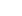Laboratoire Angevin de Recherche en Ingénierie des Systèmes

Separated by coma

Dynamic systems are systems whose state changes over time. The variation of the state of the system can be predicted using mathematical models that allow to evaluate the performances, to simulate their behaviour or to propose control strategies.
Within this group, different models of dynamic systems are considered

•     Partial derivative models, which allow the prediction of the behaviour of thermal or fluid systems,
•     discrete models to describe the evolution of systems whose state changes according to the occurrence of events, such as production, computer or transport systems.

The work carried out aims to develop new mathematical modelling tools, to use existing mathematical models to propose control strategies and to apply optimisation and operational research methods in order to design more efficient systems.
The group is also interested in the application of these tools in robotics and computer science.

Keywords: Partial differential equations, thermal systems, combinatorial optimisation, operations research, vehicle tour optimisation, discrete event systems, max-plus linear systems theory, scheduling, mobile robotics, mapping, interval analysis.